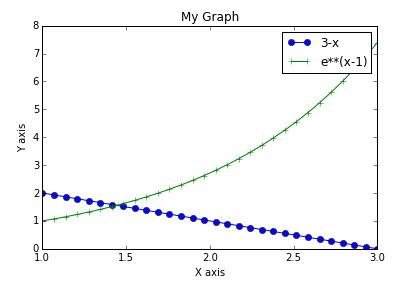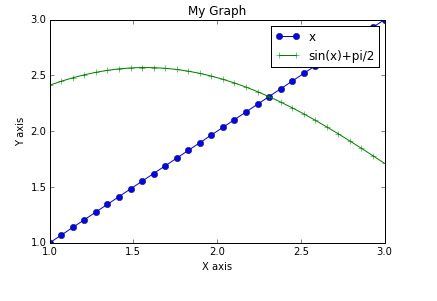### Higher Engineering Mathematics

Click to view -Chapter 1: Solution of equation and curve fittingPoint of Intersection: e^(x-1)

Click to view -Chapter 1: Solution of equation and curve fittingPoint of Intersection: sin(x)+pi/2